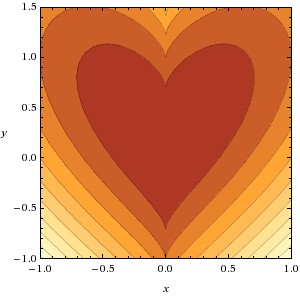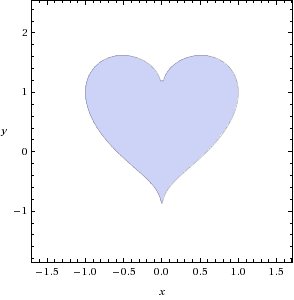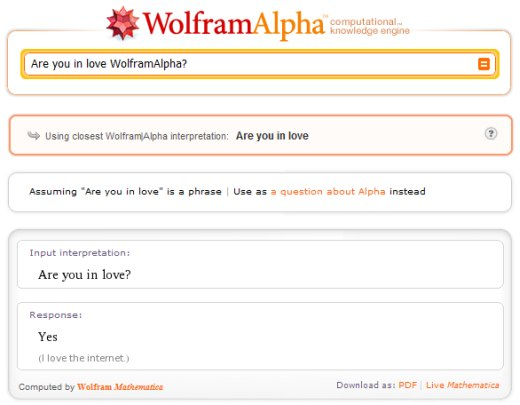# WolframAlpha in Love

So after posting that “plotting love” shirt a few days ago, a bunch of you guys started creating various equations to improve on ThinkGeek’s heart-shaped design. Here are the results, as generated by the “humane” version of Skynet itself.

plot x^2 + (y-(x^2)^(1/3))^2,x=-1..1,y=-1..1.5polar plot 2 – 2 sin t + sin t sqrt (abs(cos t))/(sin t + 1.4)plot x^2+(y-(x^2)^(1/5))^2=1plot x^2+(y-(x^2)^(1/5))^2<1And when I asked WolframAlpha if he was in love, here’s what he answered: